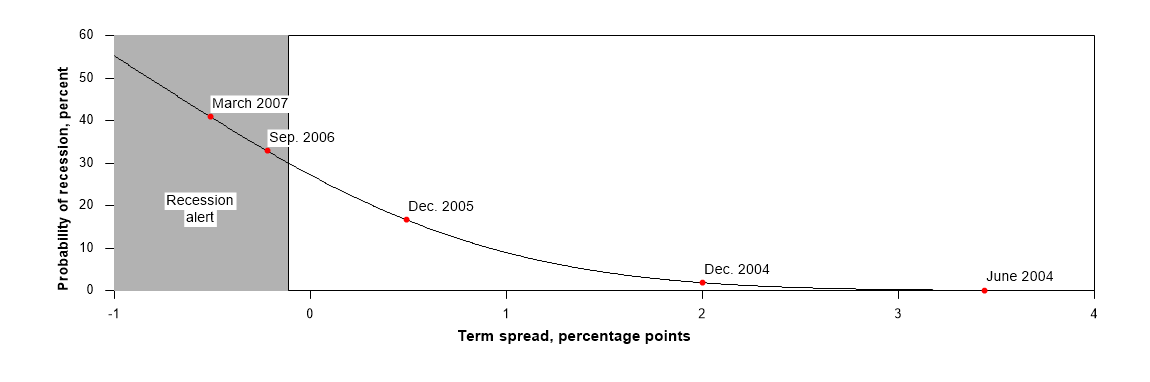Probability Estimates from the Original Model

Probability of US Recession Chart: Original Model
This section provides estimates of the probability of recession based on the original model proposed by Estrella and Hardouvelis (1989, 1991). The original model converts the monthly difference between 10-year and 3-month Treasury rates into a probability that the US economy will be in recession twelve months ahead. The model is estimated here using data for the monthly NBER recession indicator from January 1960 to twelve months prior to the end of the data sample. The final year of available data is held back in recognition of the long lags in the identification of business cycle turning points by the NBER. See Estrella and Trubin (2006) for details on the calculation of the spread and the probabilities. The following graph shows the historical performance of the original model.Monthly data for the original model (xlsx file)

Discussion
The difference between long- and short-term interest rates, known as the term spread or yield curve spread, may be used to calculate a probability that the economy will be in recession at a future date. Estrella and Hardouvelis (1989, 1991) introduced a statistical model based on the probit technique that converted the average term spread for a given quarter into a probability that the economy would be in recession four quarters later. The current application uses monthly data and calculates the probability of a recession 12 months later.

The original recession probability model, developed in early 1989 for internal use at the Federal Reserve Bank of New York, has performed well for over three decades. During that period, it has correctly forecasted all four US recessions in real time with no false positives using a threshold probability of 30% as indicator of severe risk of recession.

Conceptually, the probability of recession is based on expectations of future economic activity that are incorporated in bond prices. Adrian et al. (2019) also provide evidence that there is a causal connection between the term spread and recessions operating through the risky credit decisions of financial intermediaries.

Real-Time Tracking Before the Great Recession
Based on the original model estimated using monthly data from January 1959 to December 2004. The probability is for a recession 12 months after the observed spread, e.g., in March 2008 for March 2007.